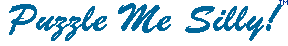On a Quest for Q's

A  C  Q  U  A  I  N  T  I  E  B  Q  H  A  I

D  E  I  F  I  L  A  U  Q  E  E  U  K  C  C

E  U  Q  S  U  R  B  U  Y  Q  Q  O  V  Q  L

L  Q  D  U  A  D  I  Q  T  E  U  T  Y  U  I

B  P  U  U  I  P  X  U  I  X  E  E  T  I  Q

B  F  Q  I  M  T  R  I  T  Q  A  I  I  T  U

A  A  R  E  T  Q  Y  E  N  U  T  U  U  L  E

U  C  N  E  U  A  L  T  A  I  H  Q  Q  I  R

Q  T  Q  O  Q  O  B  D  U  S  S  S  I  Q  U

S  Q  I  U  Q  U  E  L  Q  I  I  I  T  U  L

Q  S  U  U  I  Q  E  U  E  T  U  D  N  E  O

E  U  E  I  U  E  E  N  E  E  Q  A  A  F  U

L  N  I  A  C  A  S  S  T  I  N  U  E  I  S

T  O  T  Z  K  H  Q  C  O  P  A  Q  U  E  Y

S  E  Q  U  I  N  E  D  E  K  V  S  U  D  H

 acquaint equal querulous acquiesce equipment quiche acquit equitable quiet adequate equity quiz antiquity esq quote aqua exquisite sequined bequeath frequent squabble brusque liquefied squad clique opaque squeak disquiet qualified turquoise eloquent quantity vanquish